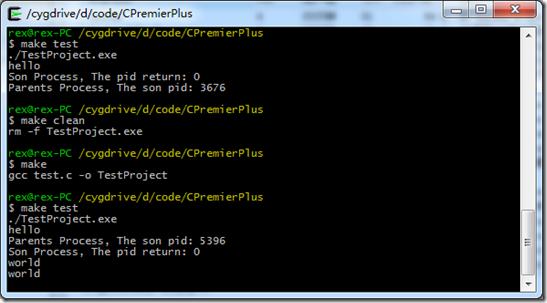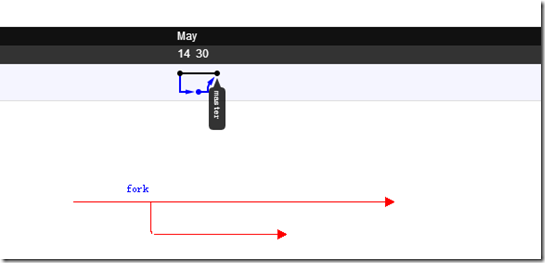fork()函数
Tag fork,进程, on by view 4922

fork函数可以复制一个当前进程。看代码

```#include <stdio.h>
int main(void){
int pid;
pid=fork();
if(pid>0){//在父进程
printf("Parents Process, The son pid: %d\n", pid);
}else if(0==pid){//在子进程
printf("Son Process, The pid return: %d\n", pid);
}else{
printf("Error!\n");
};
for(;1;){
sleep(1);
}
return 0;
}```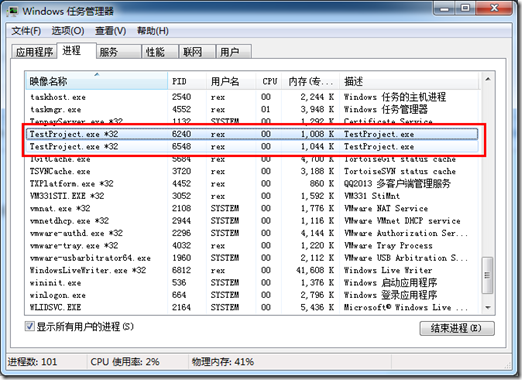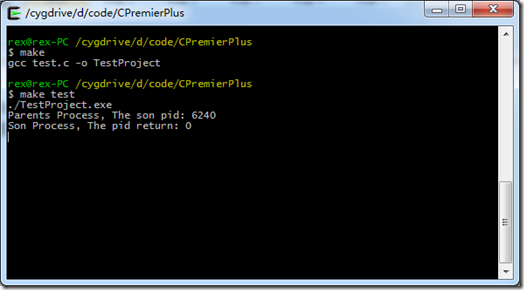```#include <stdio.h>
int main(void){
int pid;
printf("hello\n";);
pid=fork();
if(pid>0){//在父进程
printf("Parents Process, The son pid: %d\n", pid);
}else if(0==pid){//在子进程
printf("Son Process, The pid return: %d\n", pid);
}else{
printf("Error!\n");
};
for(;1;){
sleep(1);
}
return 0;
}```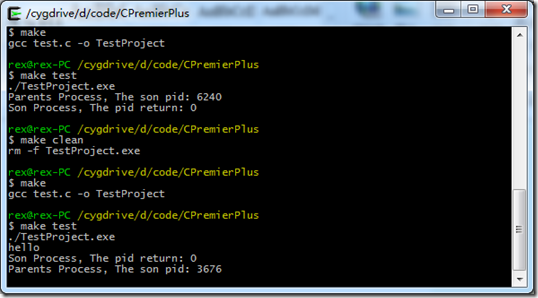```#include <stdio.h>
int main(void){
int pid;
printf("hello\n");
pid=fork();
if(pid>0){//在父进程
printf("Parents Process, The son pid: %d\n", pid);
}else if(0==pid){//在子进程
printf("Son Process, The pid return: %d\n", pid);
}else{
printf("Error!\n");
};
printf("world\n");
for(;1;){
sleep(1);
}
return 0;
}```